Function Repository Resource:

# ExponentialRegressionDataQuiz

Generate an interactive quiz for matching data to an exponential regression curve

Contributed by: Nicholas E. Brunk, Colleen Marciel F. Rosales and the Wolfram|Alpha Math Team
 ResourceFunction["ExponentialRegressionDataQuiz"][] generates an interactive quiz for users to specify points fit by a requested exponential curve.

## Details

ResourceFunction["ExponentialRegressionDataQuiz"] randomly chooses an exponential curve of best fit. The user is then tested on their ability to generate a set of points whose nonlinear regression (as determined by NonlinearModelFit) matches the requested curve. Curve parameters of the best fit curve must be within 0.5 of the requested parameters in order to be marked as correct.
Click the graph to place a data point. Right-click a given data point to remove it from the list.
The interface returned by ResourceFunction["ExponentialRegressionDataQuiz"] includes the following buttons:
 Check answer automatically grades input with feedback Reset problem resets the problem by removing all chosen points Next problem randomly generates a new problem and internally saves the previous problem history Print report prints a description of the problems that have been attempted so far, all guesses made and whether the last guess on each problem was correct

## Examples

### Basic Examples (1)

Generate an interface quiz on matching a specified regression curve:

 In:=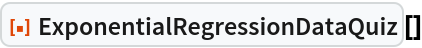Out=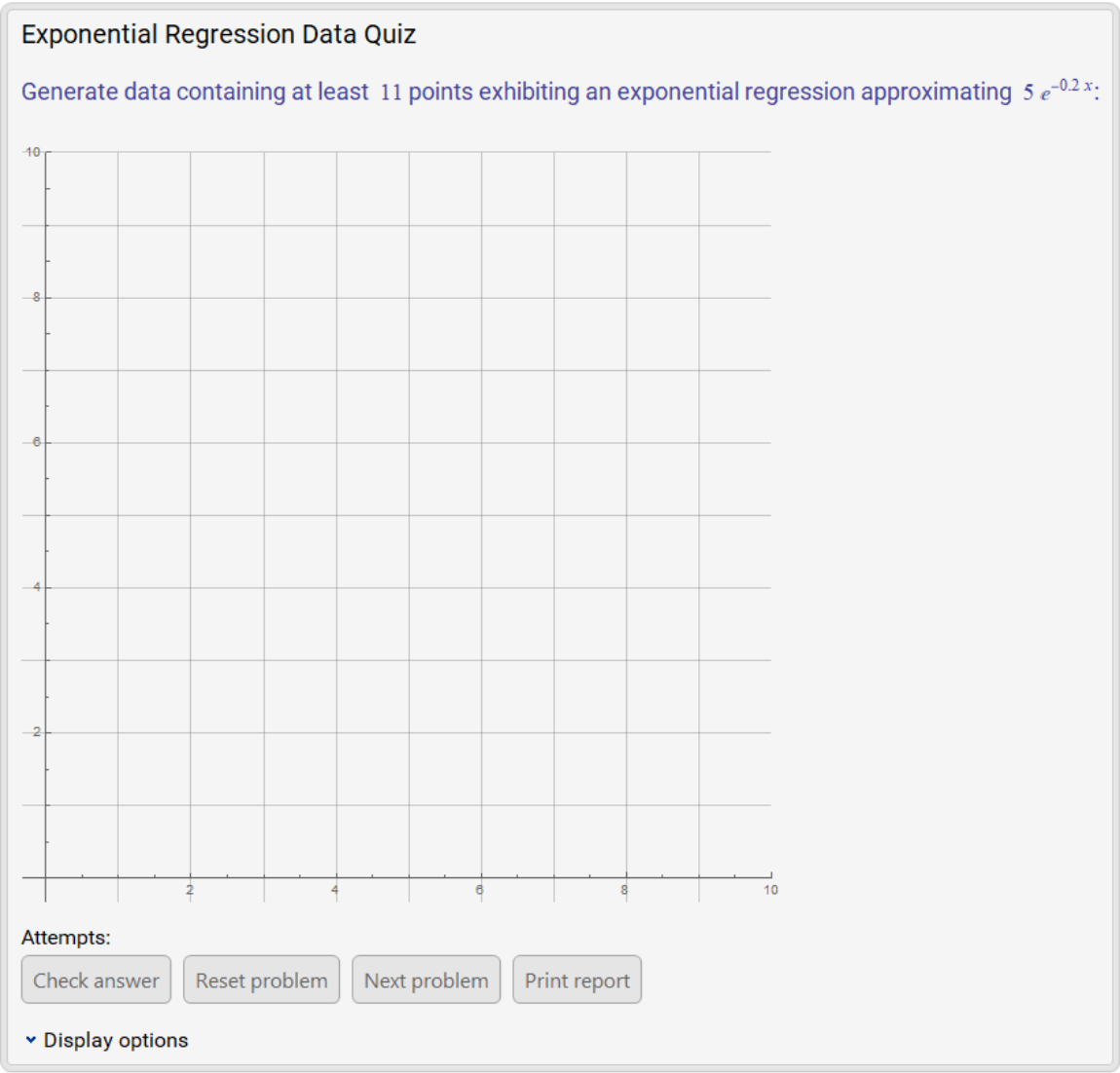### Scope (3)

Clicking the Check answer button will mark answers' correctness based on the number of points and the approximate regression curve. Correct answers receive a green check mark:

 In:=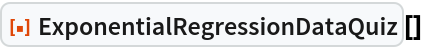Out=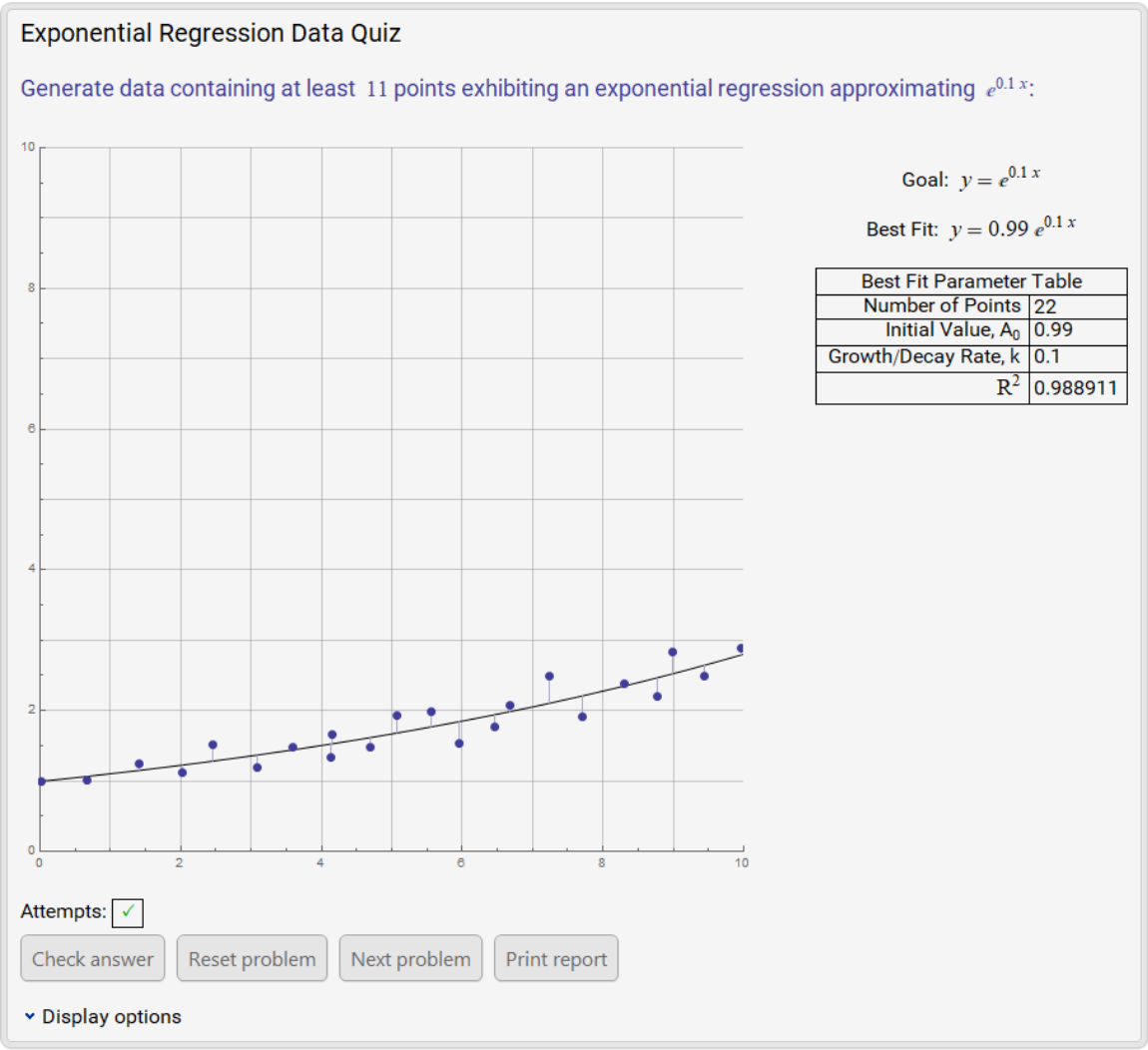If an attempt does not approximately meet the specified criteria, it is marked as incorrect with a red x:

 In:=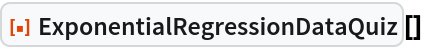Out=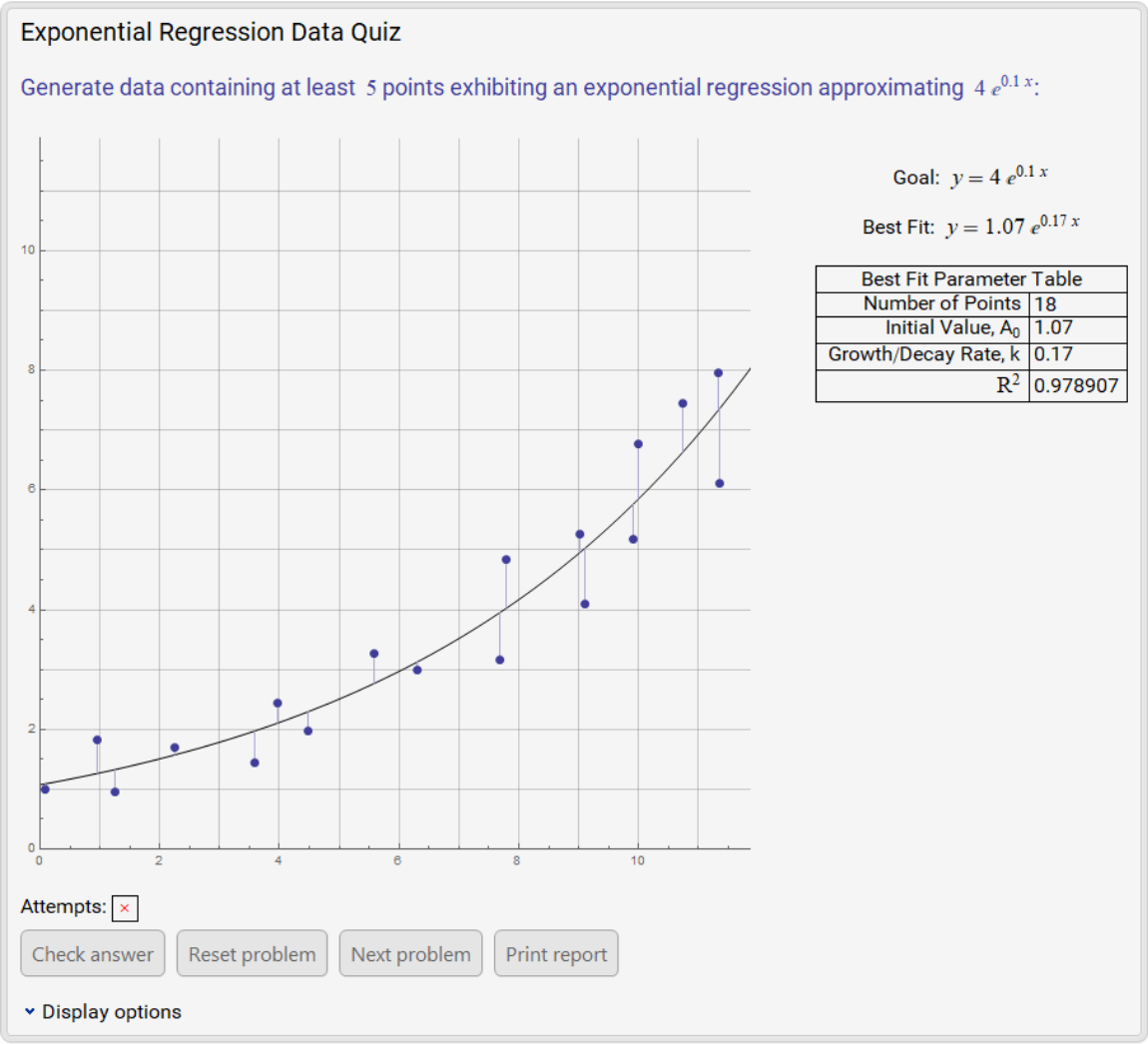Clicking the Print report button returns a description of the problems that have been attempted so far, all guesses made and if the last guess on each problem was correct:

 In:=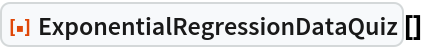Out=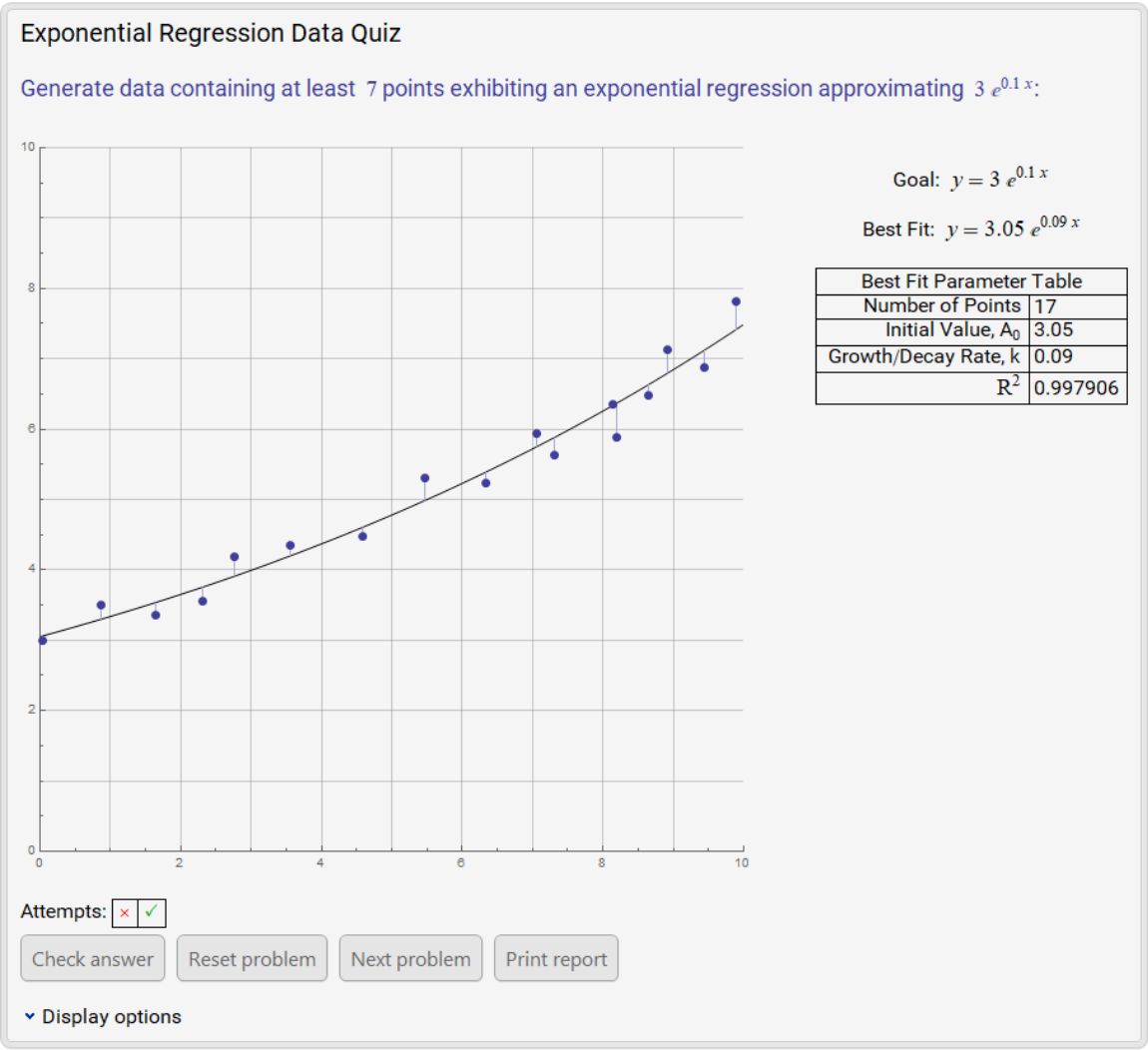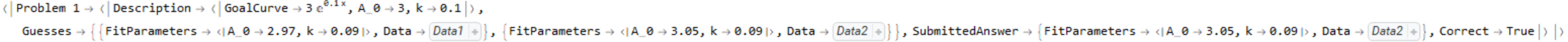## Publisher

Wolfram|Alpha Math Team

## Version History

• 2.0.0 – 23 March 2023
• 1.0.1 – 17 March 2021
• 1.0.0 – 22 February 2021

## Author Notes

To view the full source code for ExponentialRegressionDataQuiz, evaluate the following:

 In:=